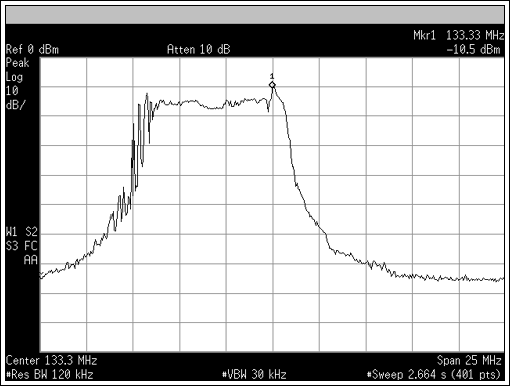# Use a Margining Oscillator as a Spread-Spectrum Clock Generator to Reduce EMI

### Abstract

This application note describes how to use a margining oscillator for applications that require spread-spectrum clock generation (SSCG). The DS4M133 margining oscillator serves as the example of the technique.

### Introduction

Margining oscillators are used to verify system operation at operating frequency extremes. The Maxim DS4M margining oscillators are controlled by a 3-state Margin Select (MS) input pin. Spread-spectrum clock generation (SSCG) is used to reduce EMI. SSCG oscillators generally integrate both the frequency control and provide a fixed amount and direction of spread. This application note discusses the use of a margining oscillator for both functions.

### Reducing EMI with a Margining Oscillator

The DS4M margining oscillators operate at the nominal frequency and at ±5%, with the frequency determined by the MS pin. When the input state is changed, the PLL tracking is nonlinear, with most of the frequency shift occurring relatively quickly. This makes the frequency at which the MS input is toggled important for achieving the best possible EMI reduction (Figures 1 and 2). The optimal frequency for the DS4M oscillators is about 8kHz, which is within the audio frequency range. Note that this frequency may be an issue in systems that encode and/or decode audio frequency signals.¹Figure 1. Clock spectrum of the DS4M133 with MS = low and MS = 8kHz (low to VCC/2) downspreading.

The data show that as the input frequency increases, the PLL response degrades. Further increases in the input frequency result in a shift away from both the fundamental frequency (fC) and the fC - 5% frequency. Due to the PLL's nonlinear response to the MS input, the maximum improvement in EMI suppression is less than the ideal case.² Since the MS input is digital and not linear, the use of modulating waveforms other than a square wave provides no benefit in EMI reduction.Figure 2. Clock spectrum of the DS4M133 with MS = 10kHz, low to VCC/2.

### Conclusion

In applications using a margining oscillator such as the DS4M series, the margining function can also be used to reduce EMI without having to use a dedicated SSCG.

References

1. Intel® technical specification, CK00 Clock Synthesizer/Driver Design Guidelines.
2. Maxim application note 3503, "Clock generation with spread spectrum."